International
Tables for
Crystallography
Volume C
Mathematical, physical and chemical tables
Edited by E. Prince

International Tables for Crystallography (2006). Vol. C, ch. 8.5, p. 708

## Section 8.5.3. Influential data points

E. Princea and C. H. Spiegelmanb

aNIST Center for Neutron Research, National Institute of Standards and Technology, Gaithersburg, MD 20899, USA, and bDepartment of Statistics, Texas A&M University, College Station, TX 77843, USA

### 8.5.3. Influential data points

| top | pdf |

Section 8.4.4discusses the influence of individual data points on the estimation of parameters and how to identify the data points that should be measured with particular care in order to make the most precise estimates of particular parameters. The same properties that cause these influential data points to be most effective in reducing the uncertainty of a parameter estimate when the model is a correct predictor for the observations also cause them to have the greatest potential for introducing bias if there is a flaw in the model or, correspondingly, if they are subject to systematic error. Reviews of procedures for studying the effects of influential data points and outliers have been given by Beckman & Cook (1983), by Chatterjee & Hadi (1986), and by Belsley (1991).

The effects of possible systematic error can be studied by identifying influential data points and then observing the effects of deleting them one by one from the refinement. The deletion of a data point should affect the standard uncertainty of an estimate, but should not cause a shift in its mean that is more than a small multiple of the resulting standard uncertainty. As in Section 8.4.4, we define the design matrix, A, by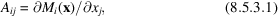whereis the model function for the ith data point, and x is a vector of adjustable parameters. Let R be the upper triangular Cholesky factor of the weight matrix, so that W = RTR, and define the weighted design matrix by Z = RA and the weighted vector of observations by y′ = Ry. The least-squares estimate of x is thenand the vector of predicted values is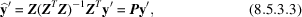where P is the projection, or hat, matrix. A diagonal element,, of P is a measure of the leverage, that is of the relative influence, of the ith data point, and therefore of the sensitivity of the estimates of the elements of x to an error in the measurement of that data point.lies in the range, and it has average value p/n, so that data points with values ofgreater than 2p/n can be considered particularly influential.

Let H = ZTZ be the normal-equations matrix, let V = H−1 be the estimated variance–covariance matrix, and let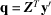, so that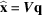. Let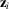be the ith row of Z, and denote by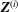,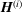,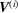,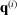, and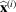the respective matrices and vectors computed with the ith data point deleted from the data set. We wish to find large values of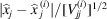, so we need to computeand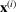. With a derivation similar to that for (8.4.4.7), it can be shown (Fedorov, 1972; Prince & Nicholson, 1985) that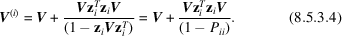Note that, if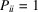, all elements ofbecome infinite, implying thatis singular. Thus, if such a data point is deleted, the solution is no longer determinate. Now,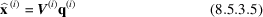and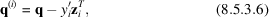so that, when V and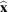have been computed once, it is a straightforward and inexpensive additional computation to determine whether any parameter has been strongly influenced, and therefore potentially biased, by the inclusion of any data point in the refinement. If there is any reason to be concerned about possible systematic error, the leverage of every data point included in the refinement should be computed, and the effects of deletion of all of those with leverage greater than 2p/n should be observed.

### References

Beckman, R. J. & Cook, R. D. (1983). Outlier..........s. Technometrics, 25, 119–149.
Belsley, D. A. (1991). Conditioning diagnostics. New York: John Wiley & Sons.
Chatterjee, S. & Hadi, A. S. (1986). Influential observations, high leverage points, and outliers in linear regression. Stat. Sci. 1, 379–393.
Fedorov, V. V. (1972). Theory of optimal experiments, translated by W. J. Studden & E. M. Klimko. New York: Academic Press.
Prince, E. & Nicholson, W. L. (1985). Influence of individual reflections on the precision of parameter estimates in least squares refinement. Structure and statistics in crystallography, edited by A. J. C. Wilson, pp. 183–195. Guilderland, NY: Adenine Press.